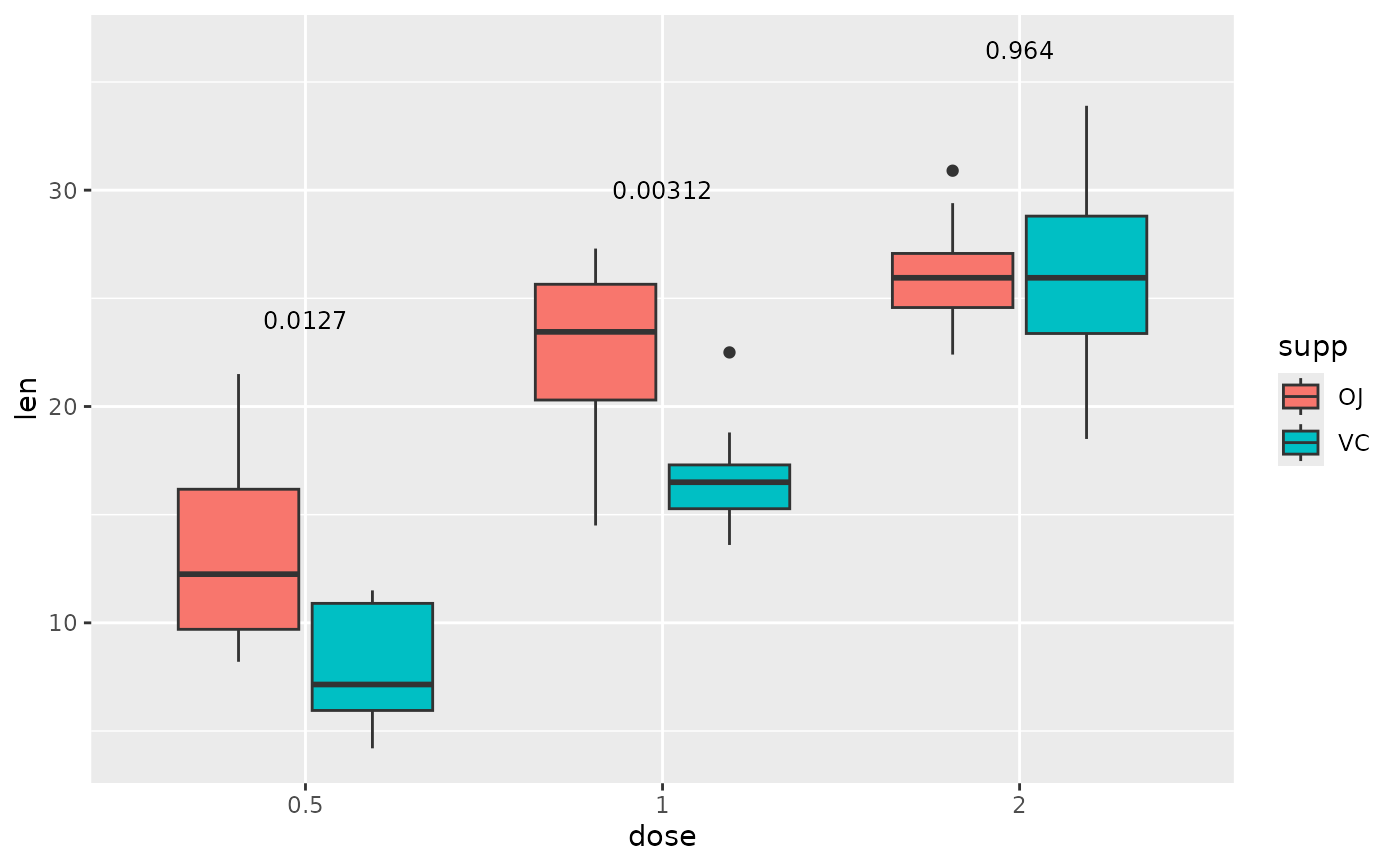Add p-values with or without brackets to a ggplot.

See here or the examples section below for examples of how to use.

add_pvalue is a refactored version of stat_pvalue_manual from kassambara/ggpubr, altered to have less dependencies, and more flexibility with input format and aesthetics. Any examples using stat_pvalue_manual found on Datanovia will also work with add_pvalue.

add_pvalue(
data,
label = NULL,
xmin = "group1",
xmax = "group2",
x = NULL,
y.position = "y.position",
parse = FALSE,
label.size = 3.2,
colour = NULL,
color = NULL,
tip.length = 0.03,
bracket.size = 0.6,
bracket.colour = NULL,
bracket.color = NULL,
bracket.shorten = 0,
bracket.nudge.y = 0,
step.increase = 0,
step.group.by = NULL,
remove.bracket = FALSE,
coord.flip = FALSE,
position = "identity",
...
)

## Arguments

data

A data.frame with the statistics to plot. Default format has the following columns: group1 | group2 | p.adj | y.position | etc. group1 and group2 are the two groups that were compared. p.adj is the adjusted p-value. y.position is the y coordinate that specifies where on the plot the p-value should go. The column names can differ from the default as long as their are specified when calling the function.

label

string. Name of column in data that contains the text to plot (e.g. label = "p.adj"). Can also be an expression that can be formatted by glue (e.g. label = "p = {p.adj}").

xmin

string. Name of column in data that contains the position of the left side of the brackets. Default is "group1".

xmax

Optional. string. Name of column in data that contains the position of the right side of the brackets. Default is "group2". If NULL, the p-values are plotted as text only.

x

string or numeric. x coordinate of the p-value text. Only use when plotting p-value as text only (no brackets).

y.position

string. Name of column in data containing the y coordinates (numeric) of each p-value to be plotted. Can also be a single number to plot all p-values at the same height or a numeric vector that will override data.

parse

logical. Default is FALSE. If TRUE the text labels will be parsed into expressions and displayed as described in ?plotmath.

label.size

numeric. Size of text.

colour, color

string. Colour of text.

tip.length

numeric vector. Length of bracket tips. Use 0 to remove tips.

bracket.size

numeric. Line width of bracket.

bracket.colour, bracket.color

string. Colour of bracket. Default is NULL which causes brackets to inherit the colour of the text.

bracket.shorten

numeric. Shortens the brackets slightly to allow them to be plotted side-by-side at the same y position.

bracket.nudge.y

numeric. Changes the y position of p-values. Useful for slightly adjusting p-values if the text is cut off.

step.increase

numeric. Changes the space between brackets.

step.group.by

string. Variable to group brackets by.

remove.bracket

logical. If TRUE all brackets are removed and p-value is shown as text only.

coord.flip

logical. If TRUE p-values are rotated by 90 degrees. Should be used with coord_flip

position

string or call to position function such as position_dodge. Typically used for adjusting x position of p-values to be in line with dodged data.

...

Additional aesthetics or arguments passed to layer. See below for allowed values.

## Value

Returns a layer ggproto object with either geom = GeomBracket or geom = GeomText.

## Allowed ... values

add_pvalue understands the following additional aesthetics or arguments:

fontface

string. Fontface of text (e.g. "bold").

fontfamily

string. Fontfamily of text (e.g. "Arial").

hjust

numeric. Horizontal justification of text.

vjust

numeric. Vertical justification of text.

alpha

numeric. Transparency of text and/or brackets.

linetype

string or numeric. Linetype of brackets (e.g. "dashed").

lineend

string. Lineend of brackets (e.g. "butt").

na.rm

logical. If FALSE (default), removes missing values with a warning. If TRUE silently removes missing values.

show.legend

logical. Should this layer be included in the legends? If NA (default), include if any aesthetics are mapped. If FALSE, never include or if TRUE, always include. It can also be a named logical vector to finely select the aesthetics to display.

inherit.aes

logical. If FALSE, overrides the default aesthetics, rather than combining with them.

## Examples

library(ggplot2)

## we will use the ToothGrowth dataset for all examples
tg <- ToothGrowth
tg$dose <- as.factor(tg$dose)
tg\$group <- factor(rep(c("grp1", "grp2"), 30))

## p-value bracket comparing two means
# p-value table (its best to use these column names)
two.means <- tibble::tribble(
~group1, ~group2, ~p,     ~y.position,
"OJ",    "VC",    0.0606, 36
)

# boxplot (or another geom...)
ggplot(tg, aes(x = supp, y = len)) +
geom_boxplot() +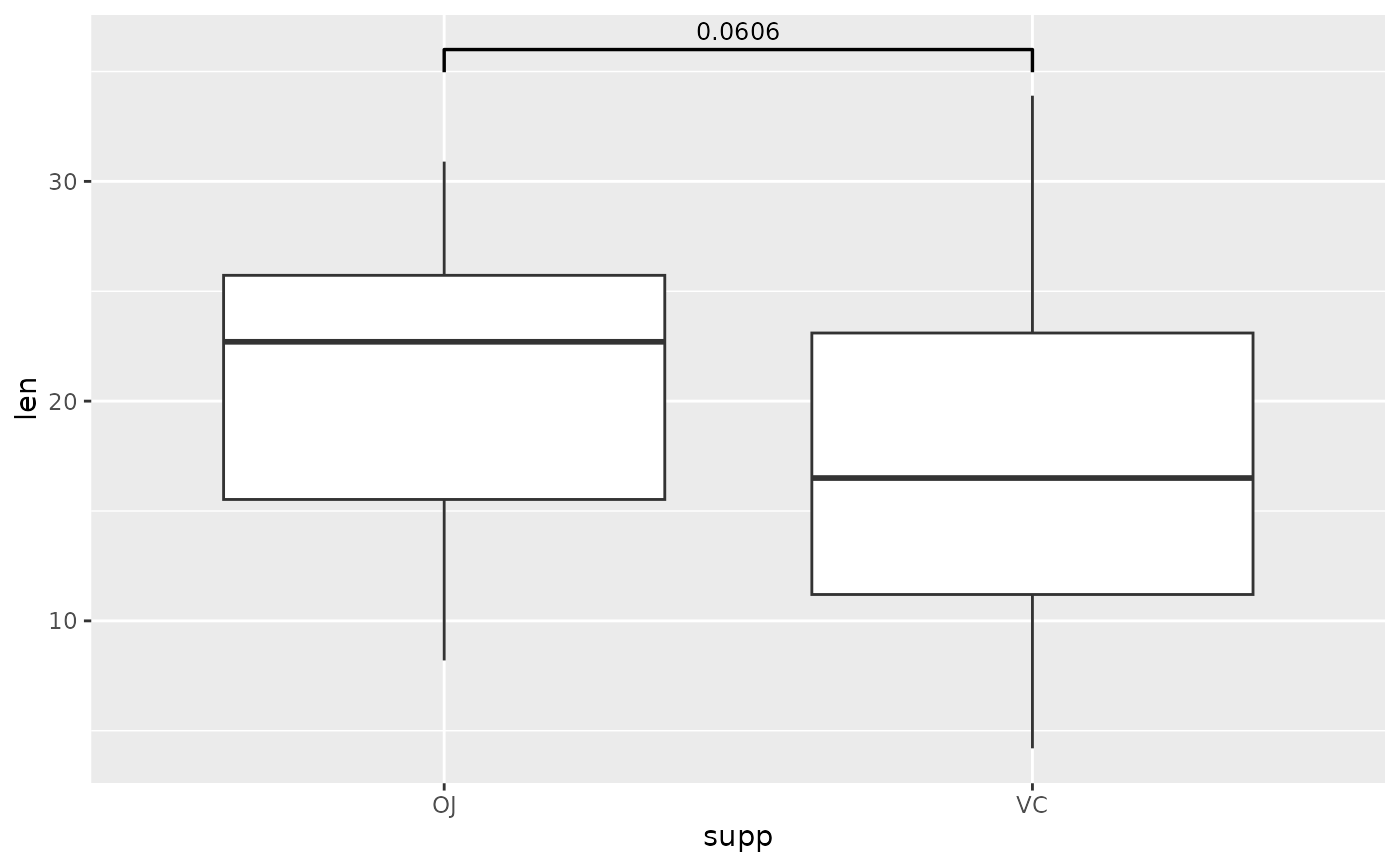# if your table has special column names you will need to specify them
two.means <- tibble::tribble(
~apple, ~banana, ~my.pval, ~some.y.position,
"OJ",    "VC",    0.0606,  36
)

ggplot(tg, aes(x = supp, y = len)) +
geom_boxplot() +
two.means,
xmin = "apple",
xmax = "banana",
label = "my.pval",
y.position = "some.y.position"
)## you can make the label a glue expression
two.means <- tibble::tribble(
~group1, ~group2, ~p,     ~y.position,
"OJ",    "VC",    0.0606, 36
)

ggplot(tg, aes(x = supp, y = len)) +
geom_boxplot() +
add_pvalue(two.means, label = "p = {p}")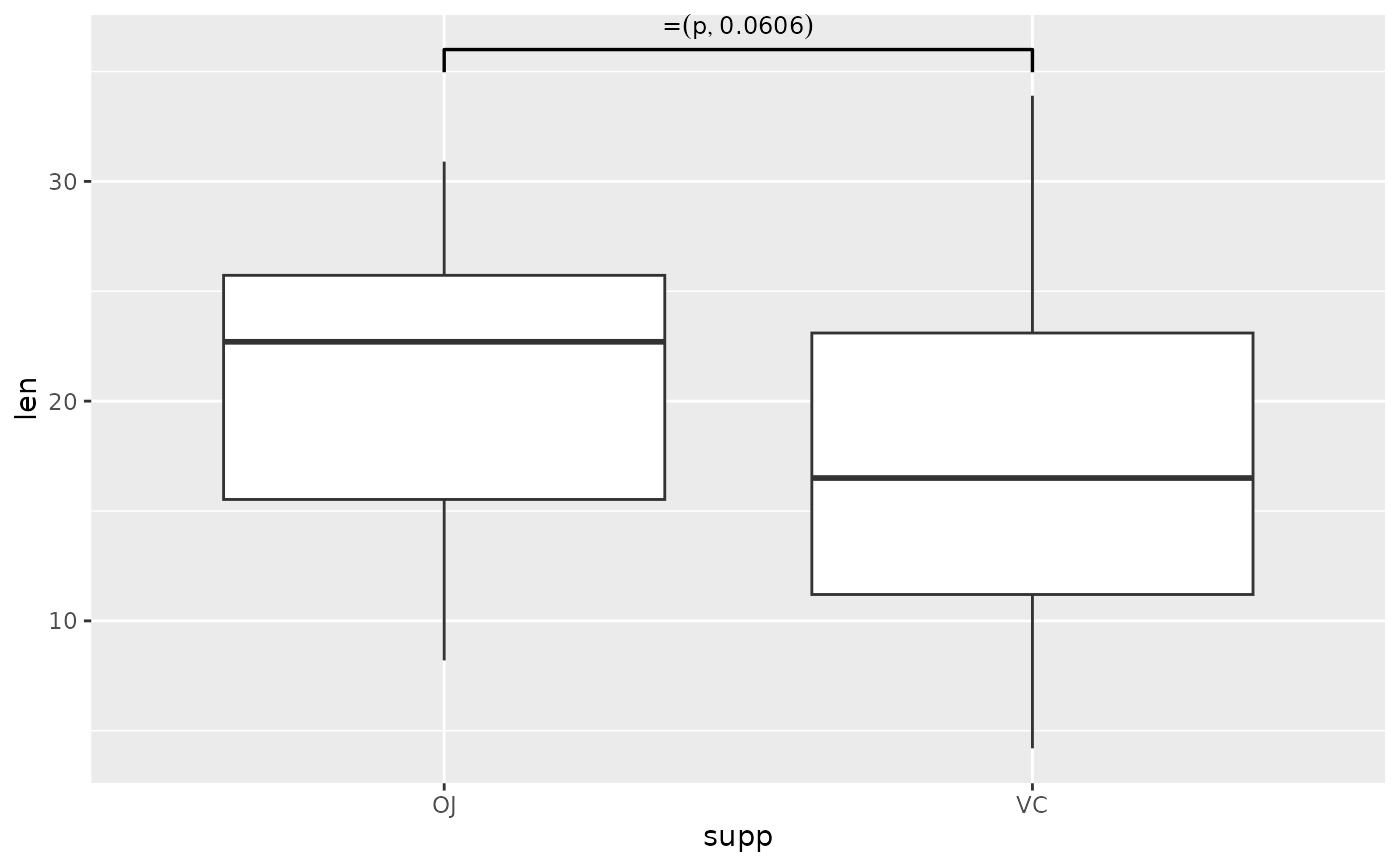## you can change aesthetics of the bracket and label
ggplot(tg, aes(x = supp, y = len)) +
geom_boxplot() +
two.means,
label = "p = {p}",
colour = "red", # label
label.size = 6, # label
fontface = "bold", # label
fontfamily = "serif", # label
angle = 45, # label
bracket.colour = "blue", # bracket
bracket.size = 1, # bracket
linetype = "dashed", # bracket
lineend = "round" # bracket
)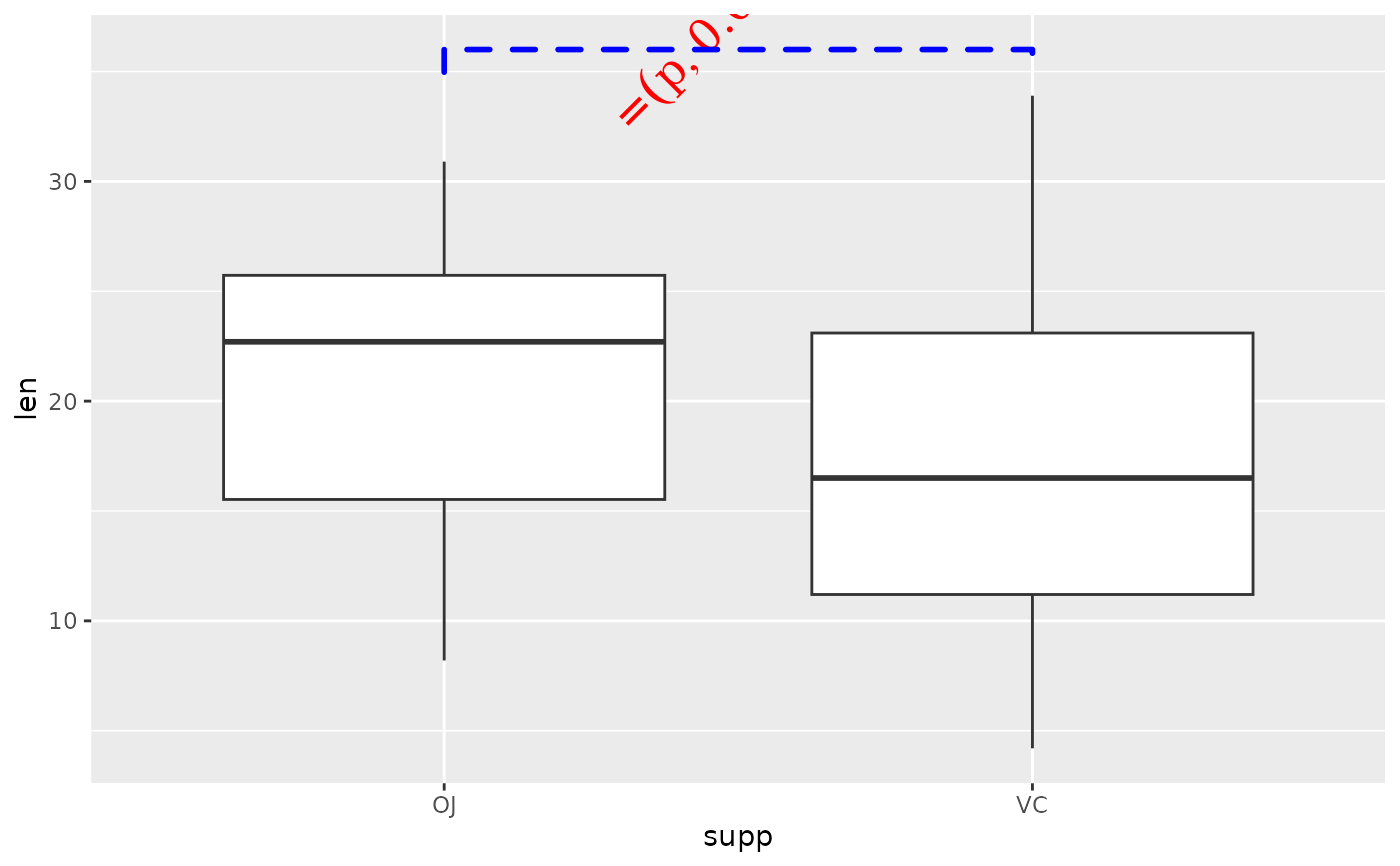## you can change the tip length of the bracket
# make them longer
ggplot(tg, aes(x = supp, y = len)) +
geom_boxplot() +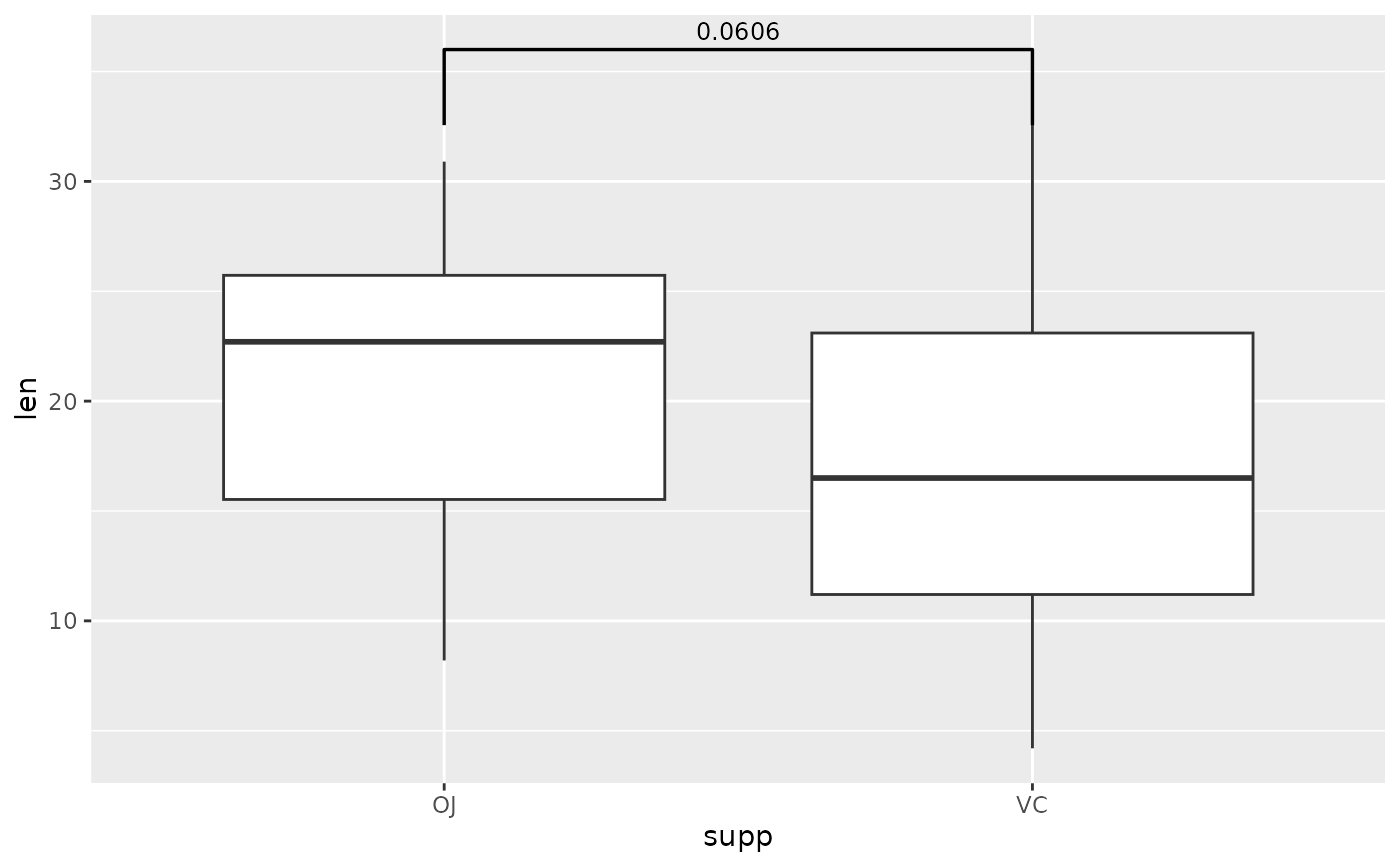# make them disappear
ggplot(tg, aes(x = supp, y = len)) +
geom_boxplot() +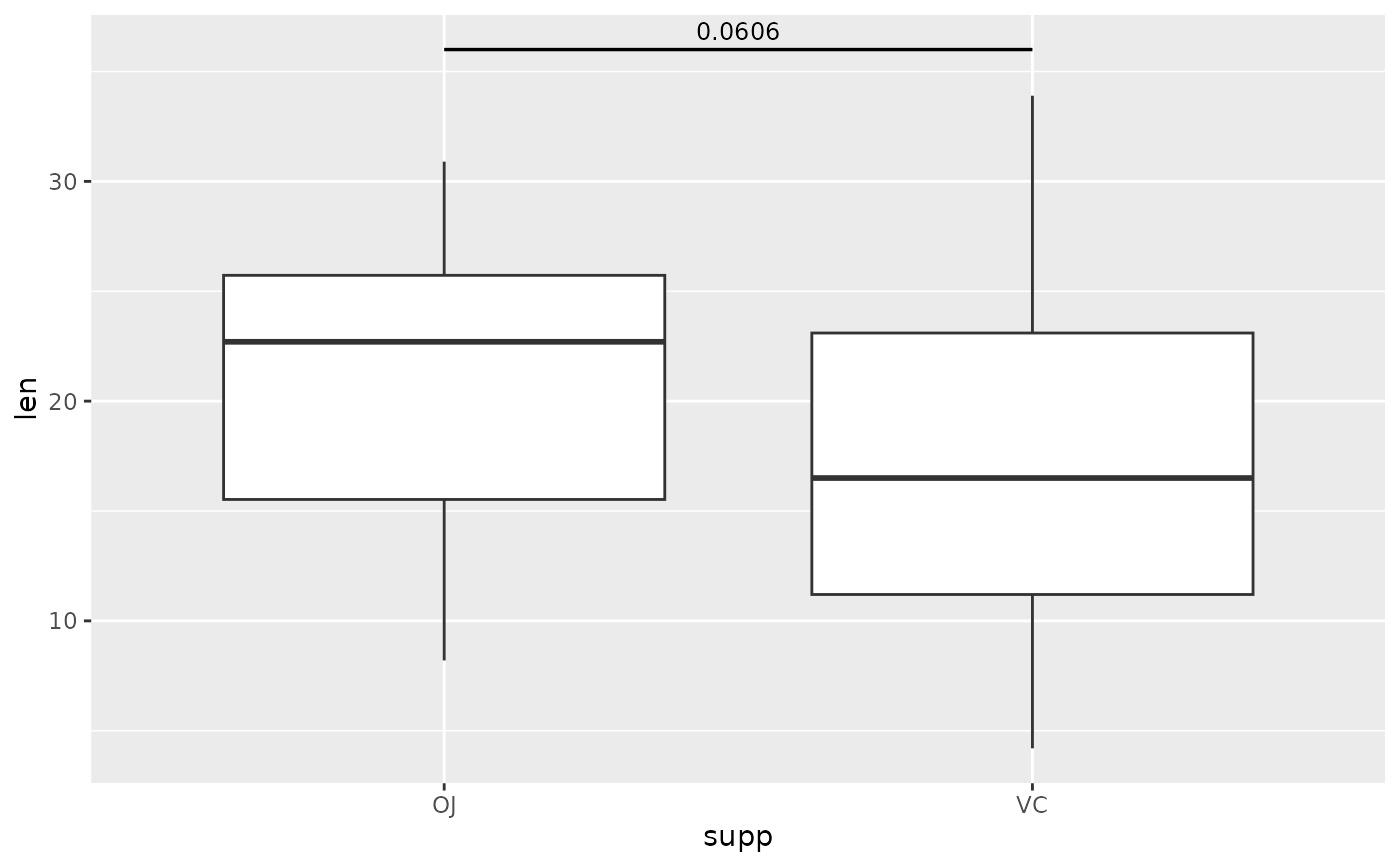# make one side longer than the other
ggplot(tg, aes(x = supp, y = len)) +
geom_boxplot() +## p-value brackets with comparisons to a reference sample
each.vs.ref <- tibble::tribble(
"0.5",   "1",     8.80e-14, 35,
"0.5",   "2",     1.27e-7,  38
)

ggplot(tg, aes(x = dose, y = len)) +
geom_boxplot(aes(fill = dose)) +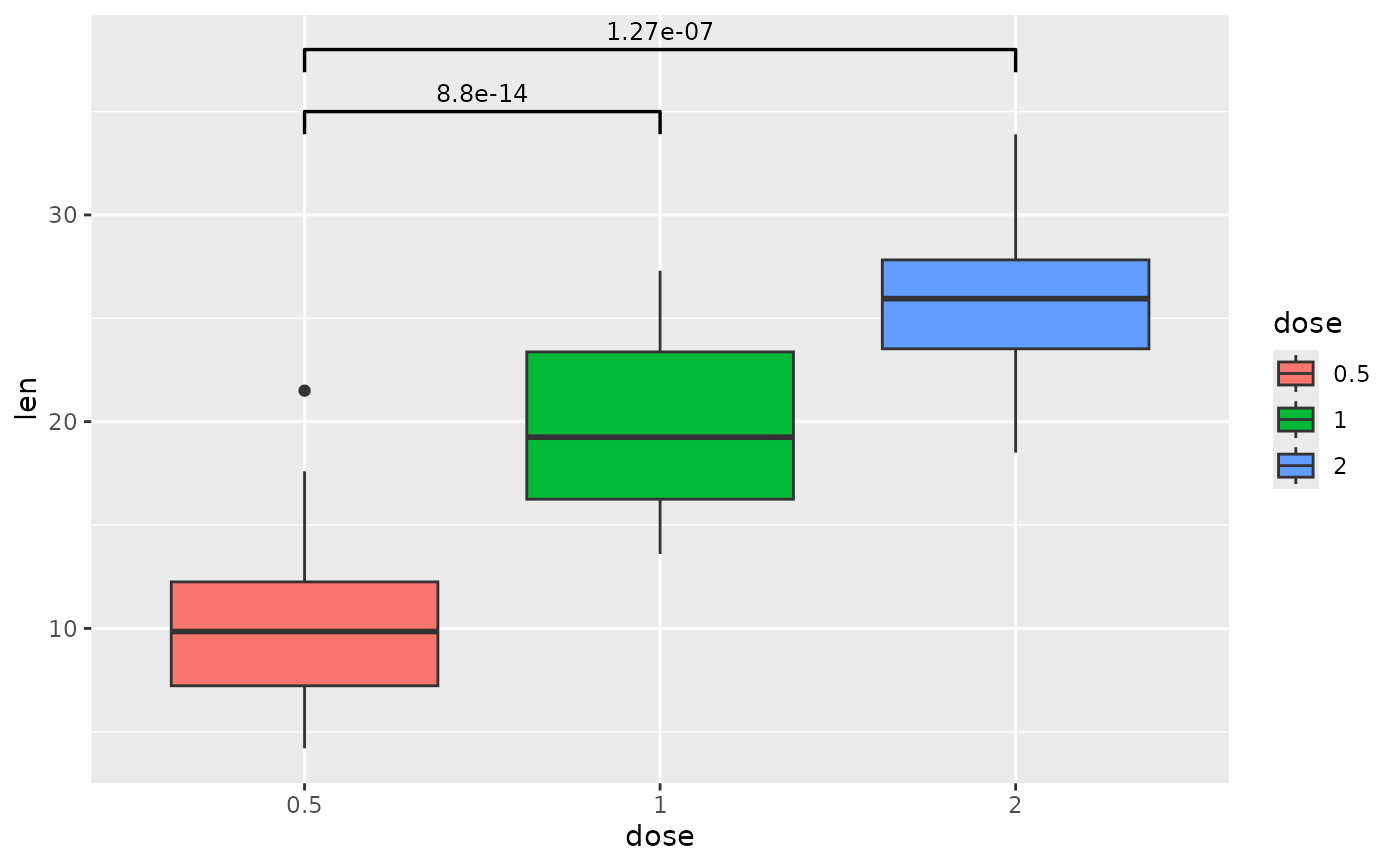## p-value brackets with pairwise comparisons
pairwise <- tibble::tribble(
~group1, ~group2,  ~p.signif, ~y.position,
"0.5",   "1",      "****",    38,
"0.5",   "2",      "****",    36,
"1",     "2",      "****",    38
)

# you can shorten the length of brackets that are close together
ggplot(tg, aes(x = dose, y = len)) +
geom_boxplot(aes(fill = dose)) +
pairwise,
bracket.shorten = c(0.05, 0, 0.05)
)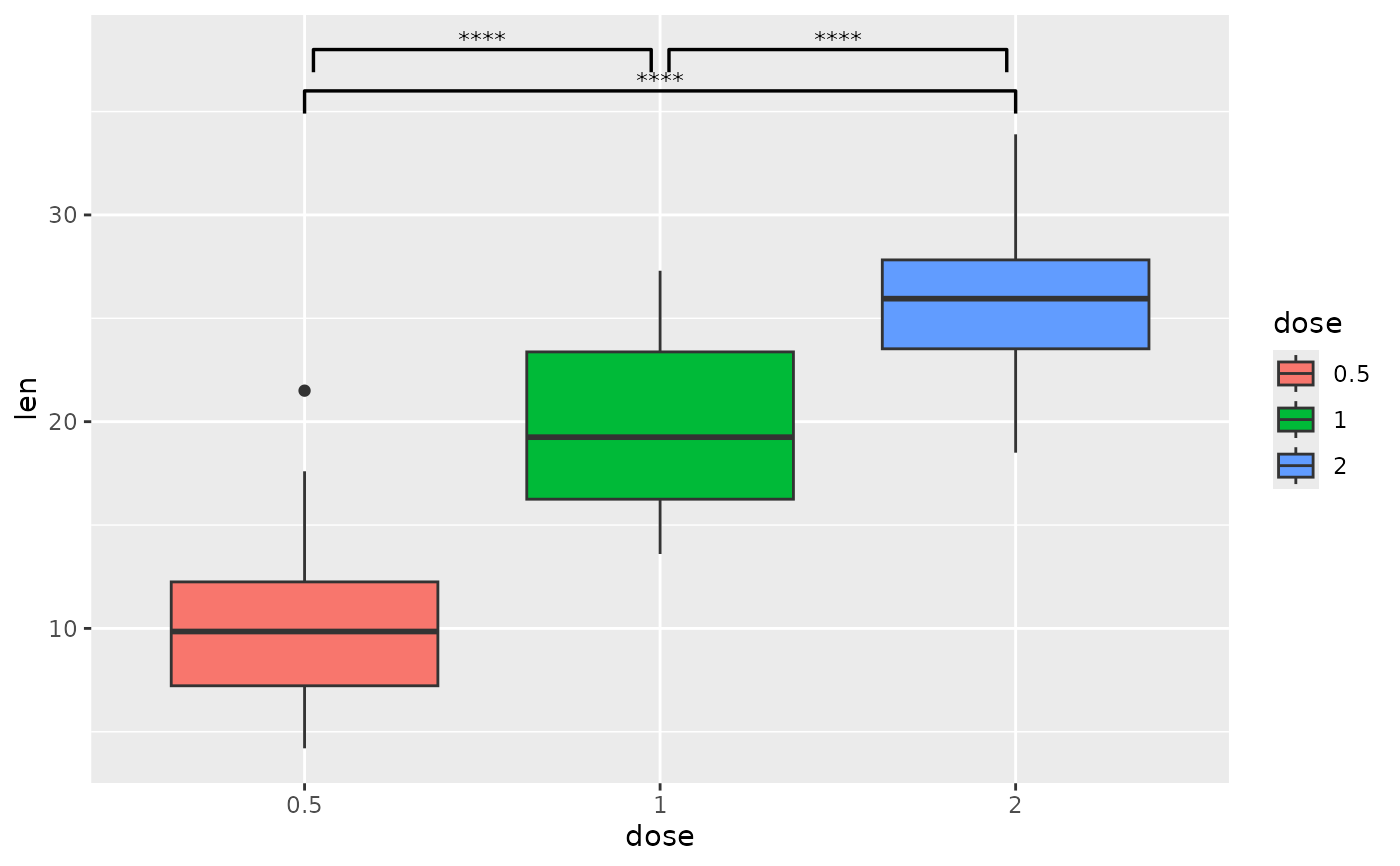# you can nudge brackets that are not quite in the correct y position
# instead of changing the p-value table
ggplot(tg, aes(x = dose, y = len)) +
geom_boxplot(aes(fill = dose)) +
pairwise,
bracket.shorten = c(0.05, 0, 0.05),
bracket.nudge.y = c(0.5, 0, 0.5)
)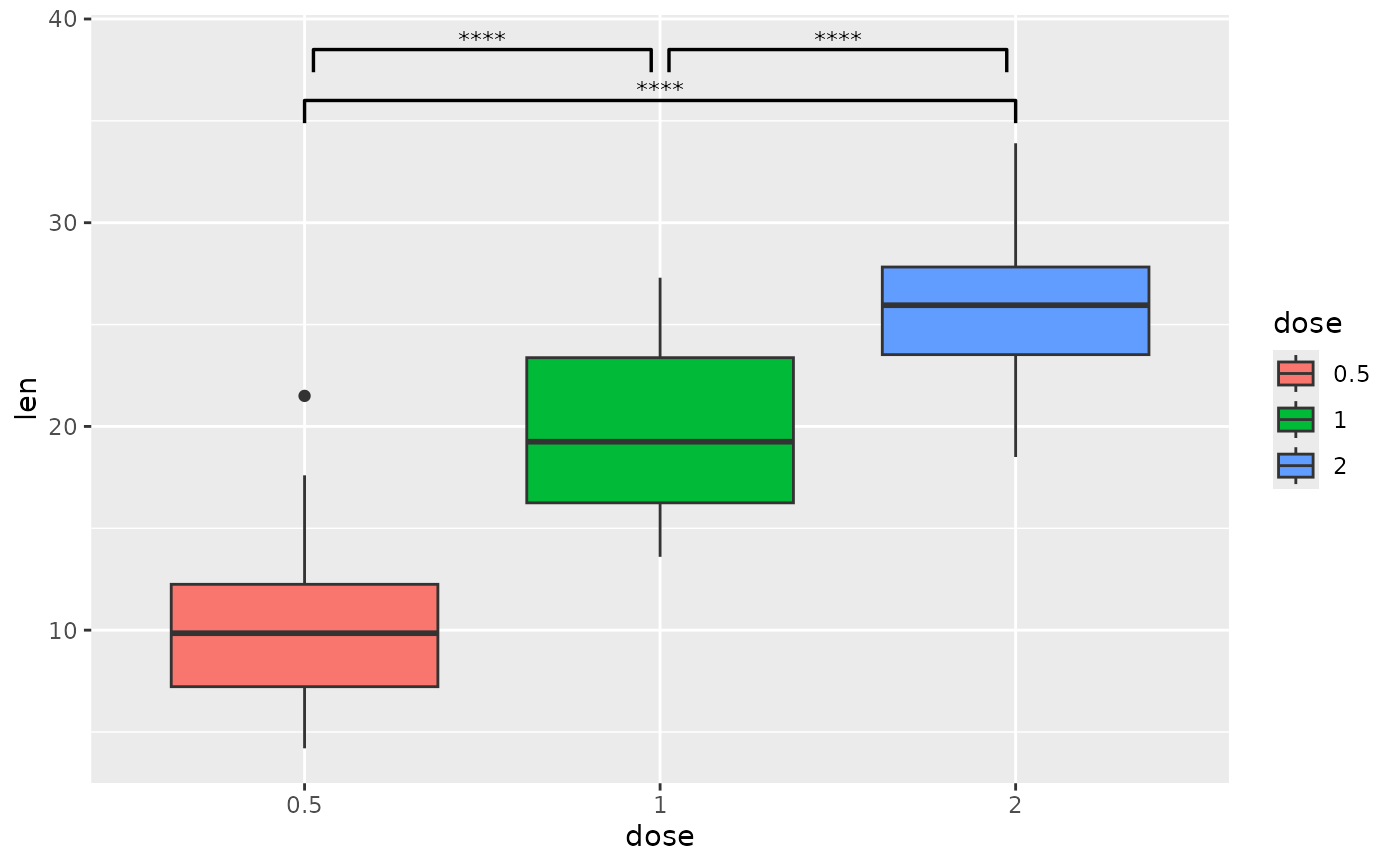## p-value brackets with pairwise comparisons of grouped data
pairwise.grouped <- tibble::tribble(
"0.5",   "1",     2.63e-4, 33.5,        "OJ",
"0.5",   "2",     3.96e-6, 37.6,        "OJ",
"1",     "2",     1.18e-1, 41.6,        "OJ",
"0.5",   "1",     2.04e-6, 36.5,        "VC",
"0.5",   "2",     1.40e-7, 40.6,        "VC",
"1",     "2",     2.75e-4, 44.6,        "VC"
)

# use step.increase to change the spacing between different brackets in the
# groups specified by step.group.by
ggplot(tg, aes(x = dose, y = len)) +
geom_boxplot(aes(fill = supp)) +
pairwise.grouped,
colour = "supp",
tip.length = 0,
step.group.by = "supp",
step.increase = 0.03
)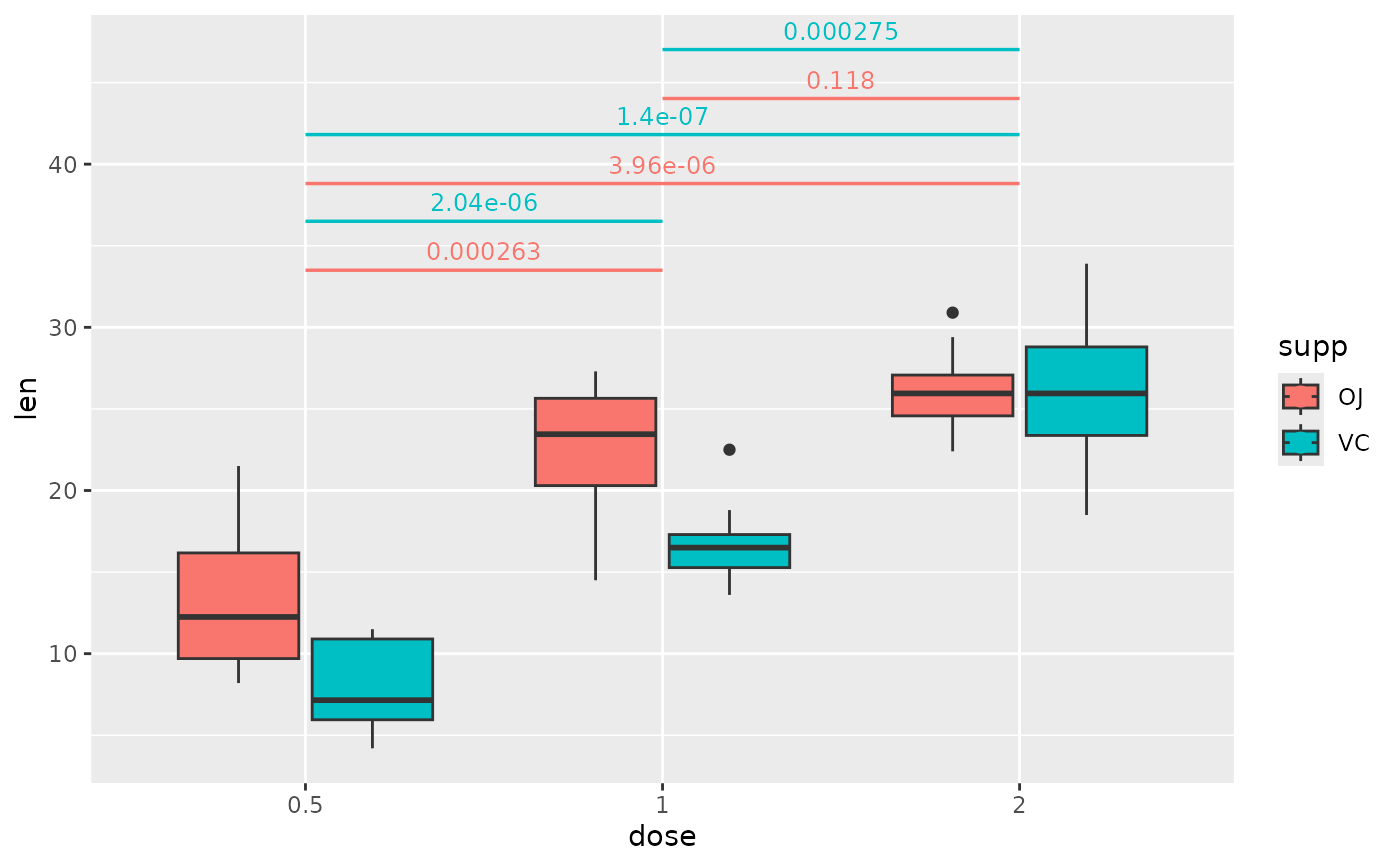## p-value (brackets) with single facet variable
two.means.grouped1 <- tibble::tribble(
"OJ",    "VC",    0.0127,  24,          "0.5",
"OJ",    "VC",    0.00312, 30,          "1",
"OJ",    "VC",    0.964,   36.5,        "2"
)

ggplot(tg, aes(x = supp, y = len)) +
geom_boxplot() +
facet_wrap(~ dose, scales = "free") +
add_pvalue(two.means.grouped1) # table must have dose column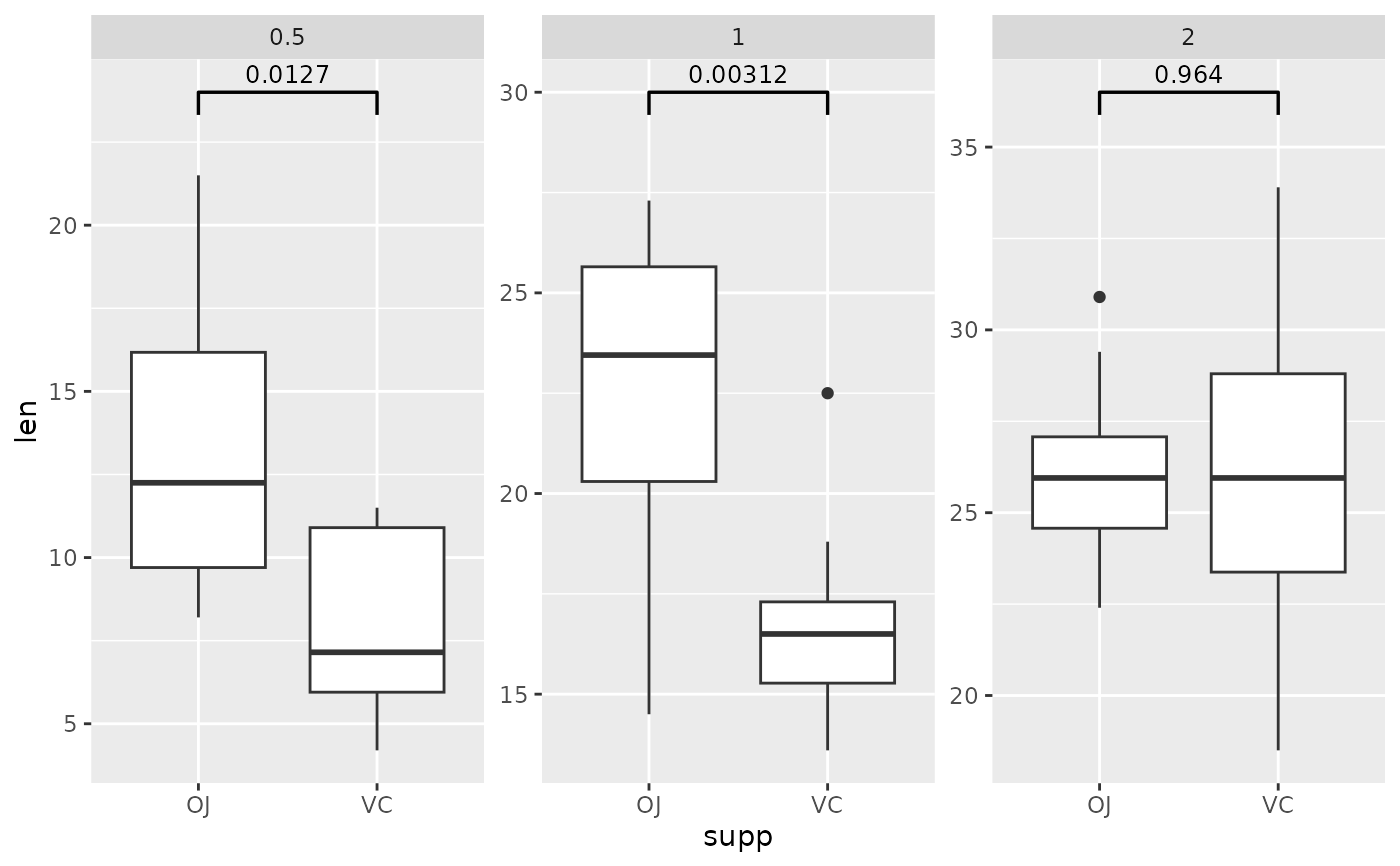## p-value (brackets) with single facet variable and multiple brackets per facet
pairwise.grouped <- tibble::tribble(
"0.5",   "1",     2.63e-4, 33.5,        "OJ",
"0.5",   "2",     3.96e-6, 37.6,        "OJ",
"1",     "2",     1.18e-1, 41.6,        "OJ",
"0.5",   "1",     2.04e-6, 36.5,        "VC",
"0.5",   "2",     1.40e-7, 40.6,        "VC",
"1",     "2",     2.75e-4, 44.6,        "VC"
)

ggplot(tg, aes(x = dose, y = len)) +
geom_boxplot(aes(fill = supp)) +
facet_wrap(~ supp) +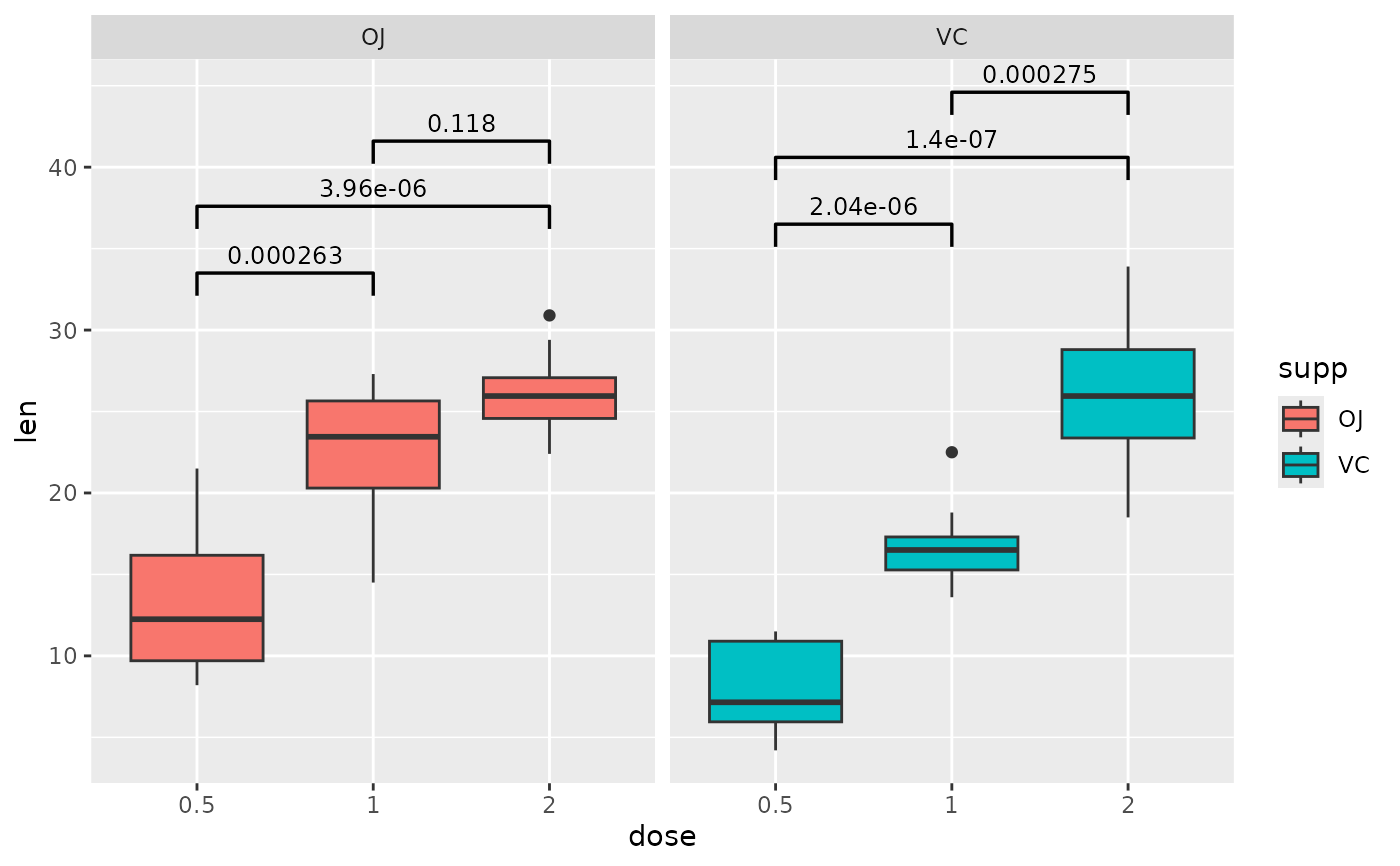## p-value (brackets) with two facet variables
two.means.grouped2 <- tibble::tribble(
~group1, ~group2, ~p.signif, ~y.position, ~group, ~dose,
"OJ",    "VC",    "*",       21,          "grp1", "0.5",
"OJ",    "VC",    "**",      30,          "grp2", "1"
)

ggplot(tg, aes(x = supp, y = len)) +
geom_boxplot() +
facet_wrap(group ~ dose) +
add_pvalue(two.means.grouped2) # table must have dose and group column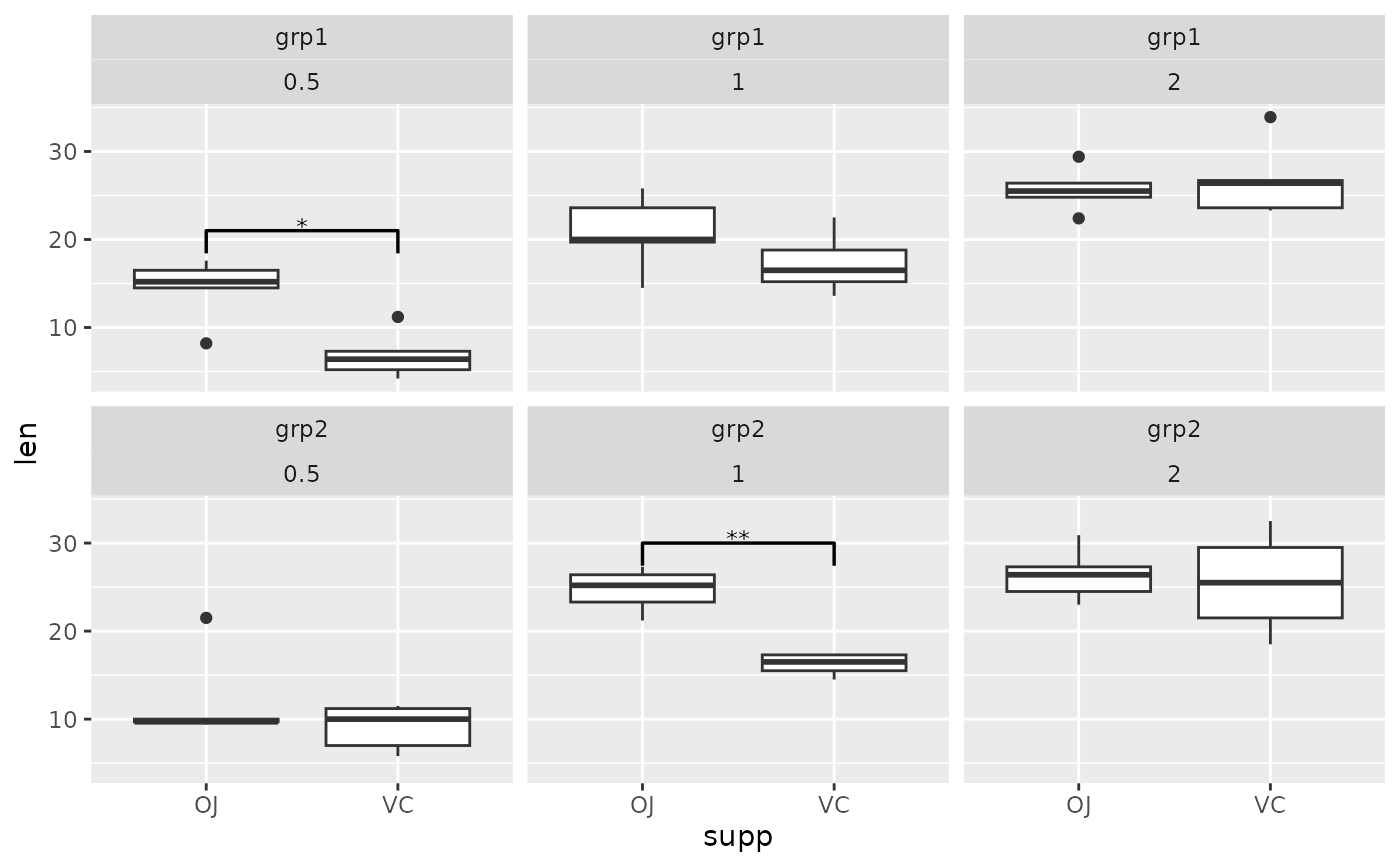## p-value (text only) comparing two means
two.means <- tibble::tribble(
~group1, ~group2, ~p,     ~y.position,
"OJ",    "VC",    0.0606, 36
)

ggplot(tg, aes(x = supp, y = len)) +
geom_boxplot() +
add_pvalue(two.means, remove.bracket = TRUE, x = 1.5)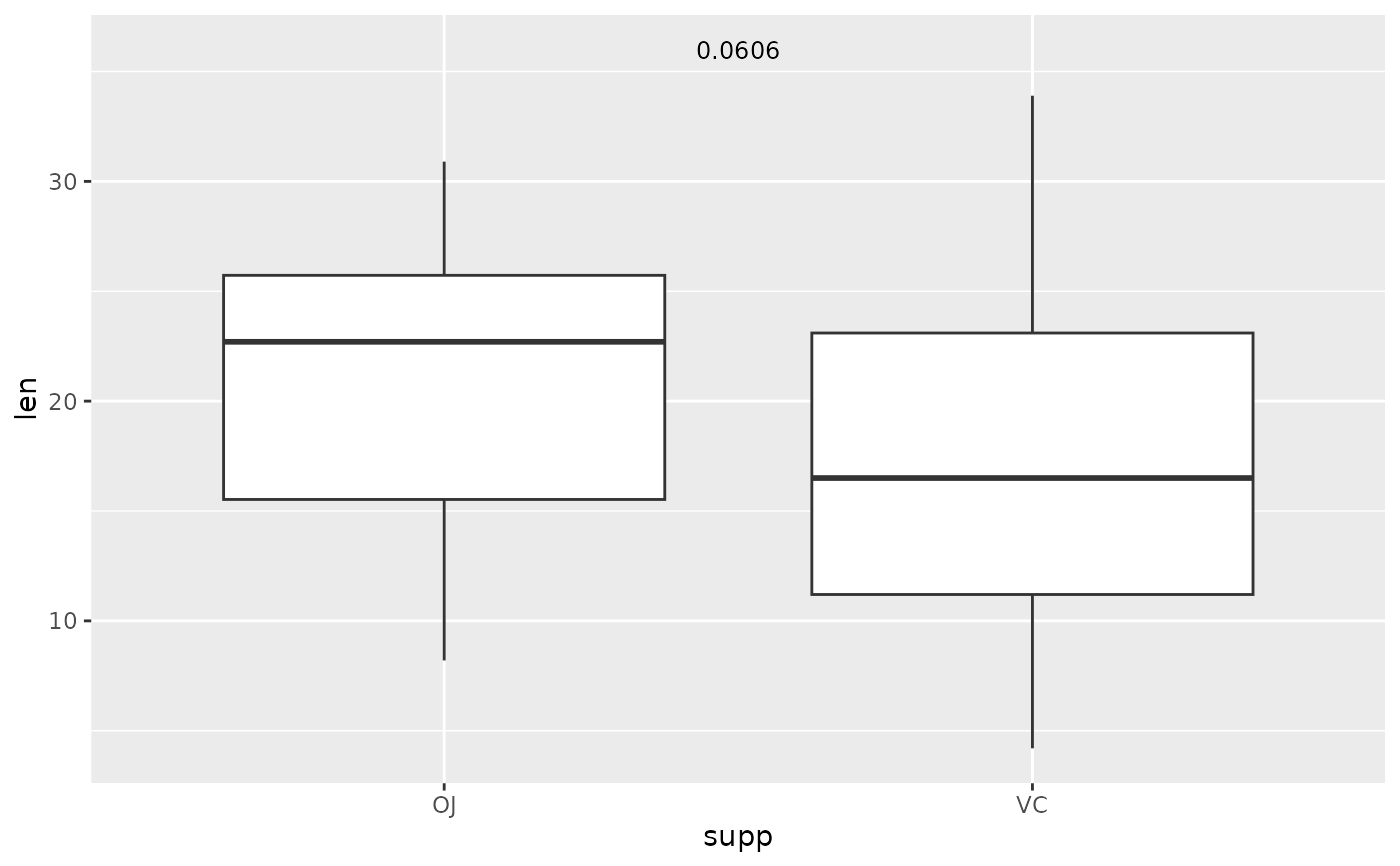## p-value (text only) with coord_flip, override y.position, change angle
ggplot(tg, aes(x = supp, y = len)) +
geom_boxplot() +
two.means,
remove.bracket = TRUE,
x = 1.5,
y.position = 32,
angle = 45
) +
coord_flip()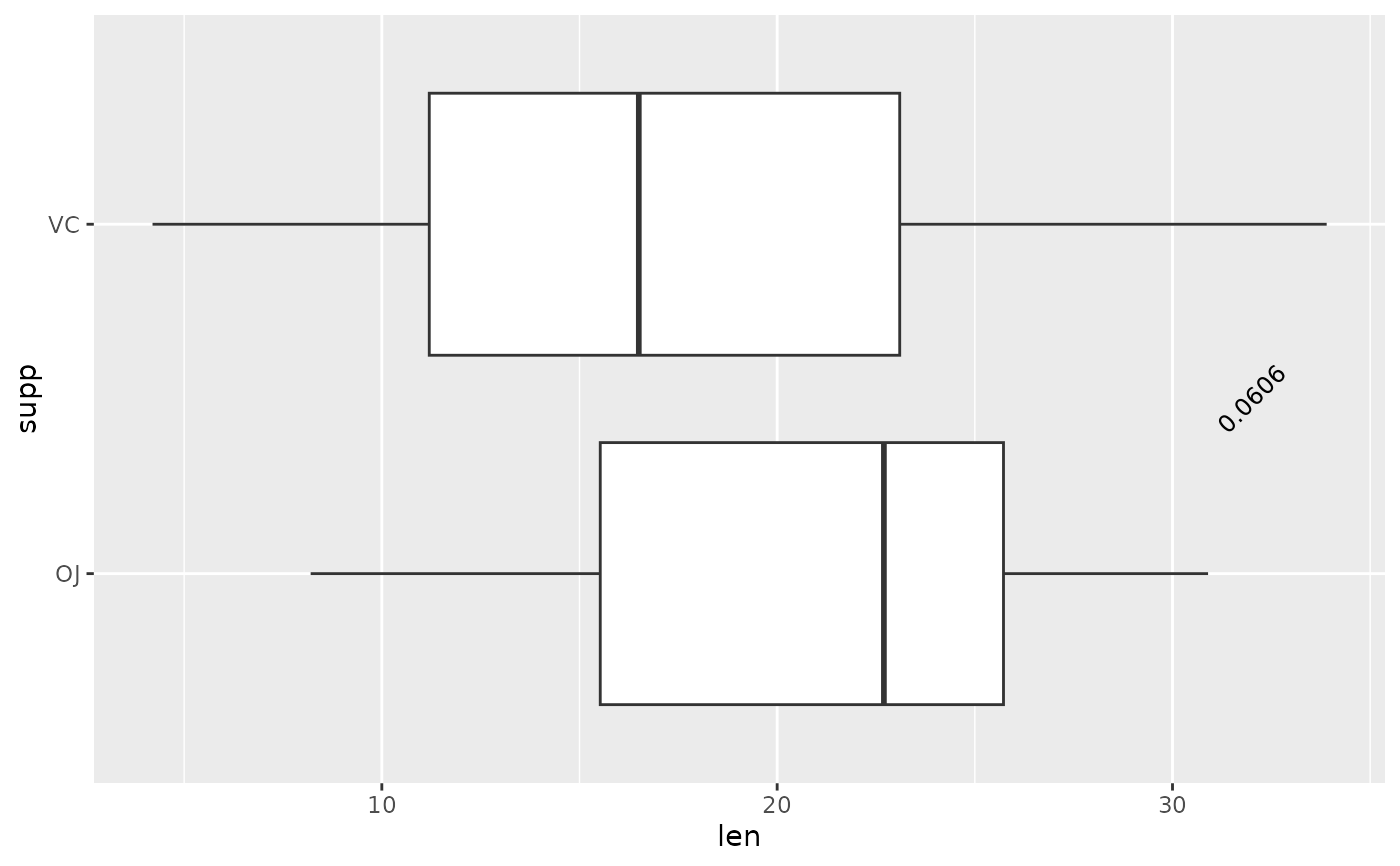## p-value (text only) comparing to the null
one.mean <- tibble::tribble(
~group1, ~group2,      ~p.signif, ~y.position, ~dose,
"1",     "null model", "****",    35,          "0.5",
"1",     "null model", "****",    35,          "1",
"1",     "null model", "****",    35,          "2"
)

ggplot(tg, aes(x = dose, y = len)) +
geom_boxplot(aes(fill = dose)) +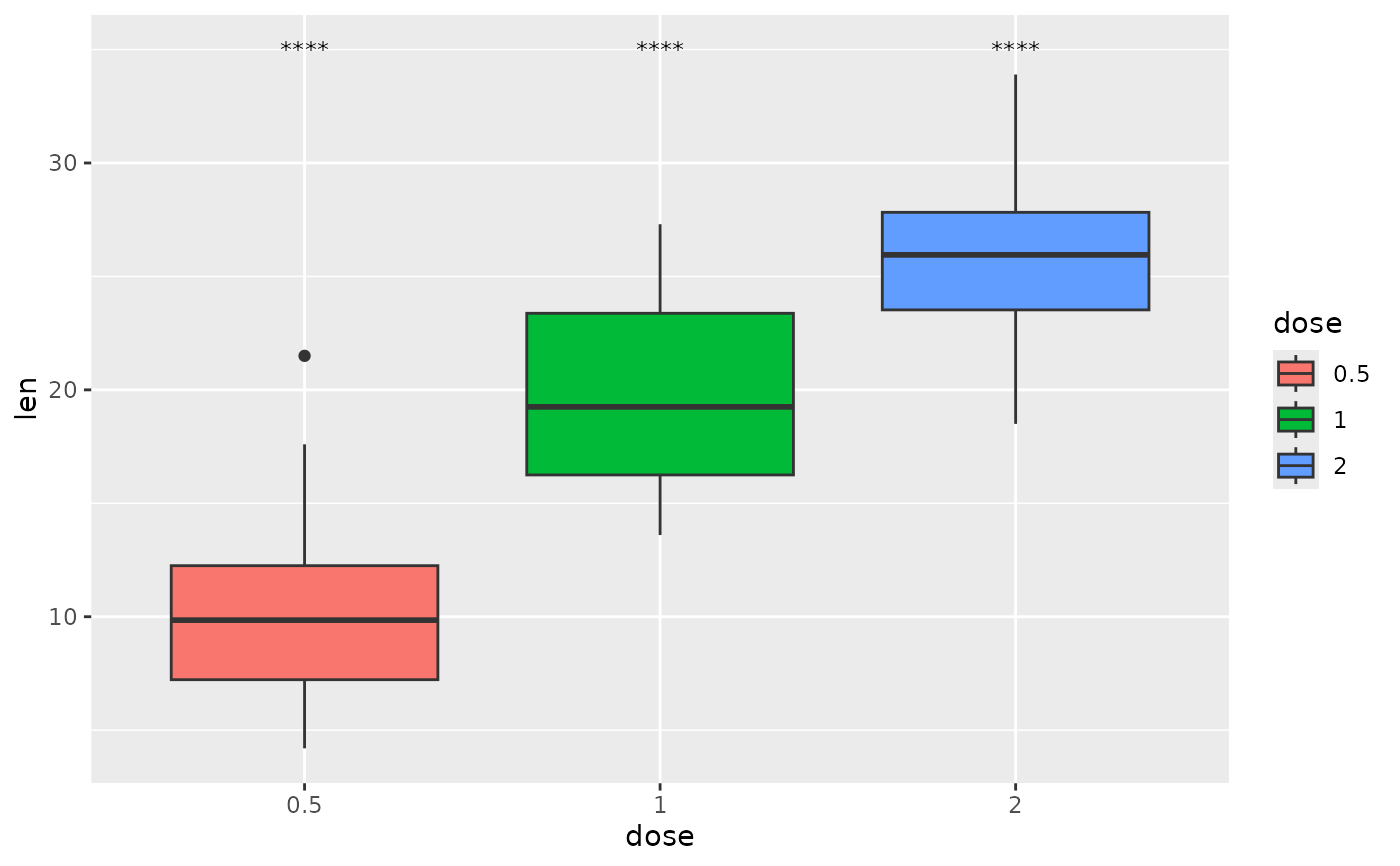## p-value (text only) with comparisons to a base mean
each.vs.basemean <- tibble::tribble(
"all",   "0.5",   "****", 35,
"all",   "1",     "ns",   35,
"all",   "2",     "****", 35
)

ggplot(tg, aes(x = dose, y = len)) +
geom_boxplot(aes(fill = dose)) +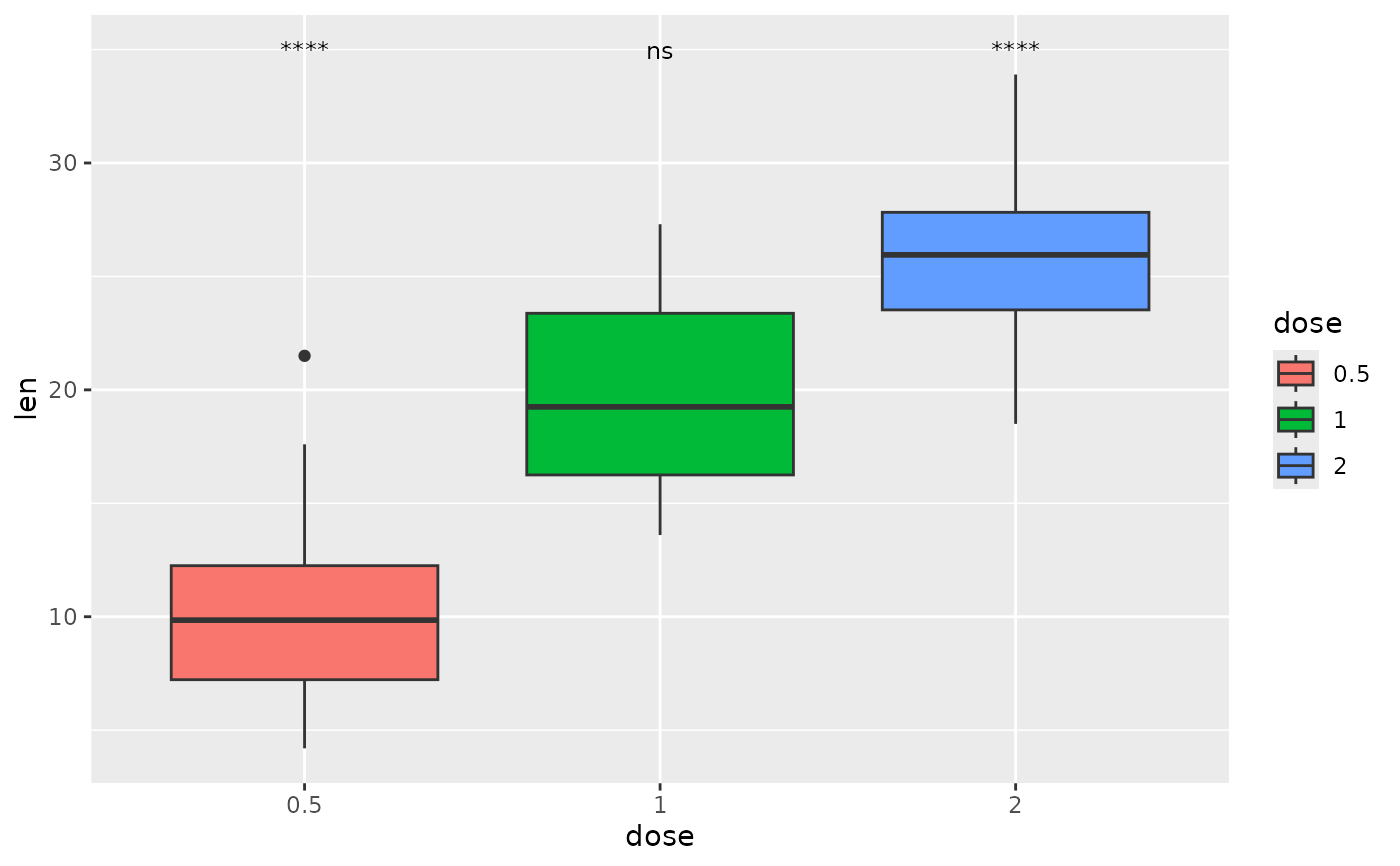## p-value (text only) with comparison to reference sample
each.vs.ref <- tibble::tribble(
"0.5",   "1",     8.80e-14, 35,
"0.5",   "2",     1.27e-7,  38
)

ggplot(tg, aes(x = dose, y = len)) +
geom_boxplot(aes(fill = dose)) +
add_pvalue(each.vs.ref, coord.flip = TRUE, remove.bracket = TRUE)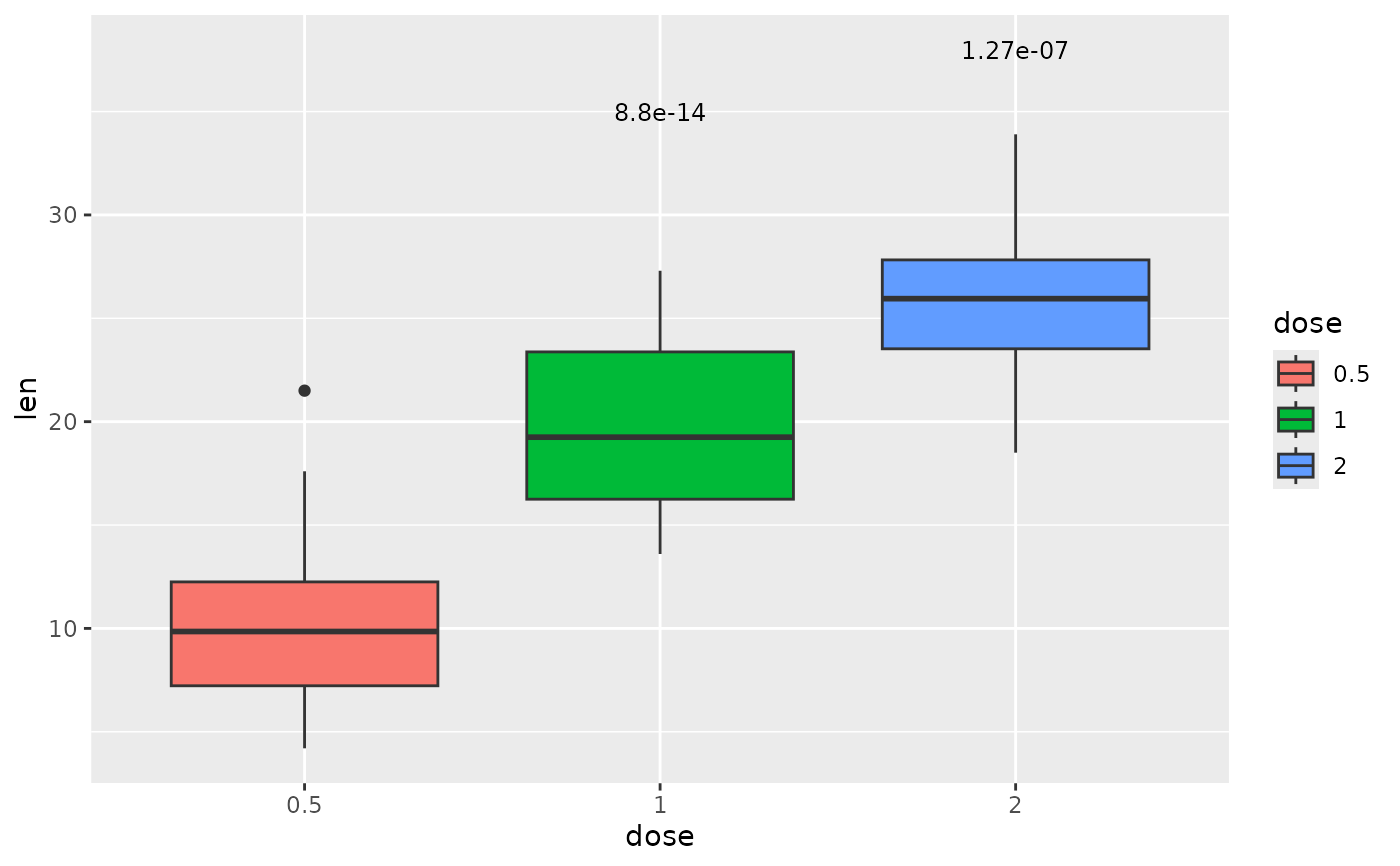## p-value (text only) with a grouping variable
two.means.grouped1 <- tibble::tribble(